# Algebra 1 : Functions and Lines

## Example Questions

### Example Question #53 : How To Find The Midpoint Of A Line Segment

Two points,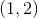and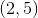, are connected to form a line.  What is the midpoint?

Possible Answers: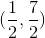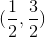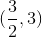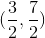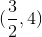Correct answer:Explanation:

Write the midpoint formula.  The midpoint is the center of the line segment.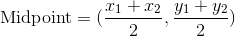Substitute the values of the coordinates.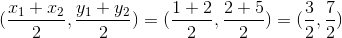The midpoint is:### Example Question #55 : How To Find The Midpoint Of A Line Segment

Find the midpoint of the line segment formed by the points

(-1, -3) and (3, 3).

Possible Answers:

(2, 2)

(2, 0)

(1, 0)

(-4, -6)

(1, -1)

Correct answer:

(1, 0)

Explanation:

To find the midpoint between two points, we use the following formula: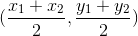where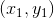and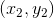are the points given.  So, given the points

(-1, -3) and (3, 3), we can substitute into the formula.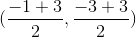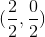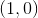Therefore, the midpoint between (-1, -3) and (3, 3) is (1, 0).

### Example Question #54 : How To Find The Midpoint Of A Line Segment

Find the midpoint of a line segment with the following endpoints: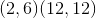Possible Answers: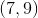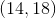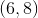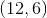Correct answer:Explanation:

Finding the midpoint of a line segment only requires that we find the midpoint, or average of both the x and y components. In order to do that, we use the following formula:For the points (2,6) and (12,12) plug in the numbers and solve: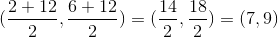This gives a final answer of### Example Question #51 : How To Find The Midpoint Of A Line Segment

Find the midpoint of the line segment with the following endpoints: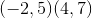Possible Answers: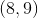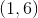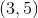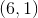Correct answer:Explanation:

Finding the midpoint of a line segment only requires that we find the midpoint, or average of both the x and y components. In order to do that, we use the following formula:For the points (-2,5) and (4,7) plug in the numbers and solve: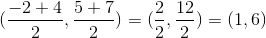This gives a final answer of### Example Question #55 : How To Find The Midpoint Of A Line Segment

Find the midpoint of the line segment with the following endpoints: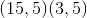Possible Answers: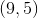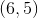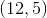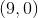Correct answer:Explanation:

Finding the midpoint of a line segment only requires that we find the midpoint, or average of both the x and y components. In order to do that, we use the following formula:For the points (15,5) and (3,5) plug in the numbers and solve: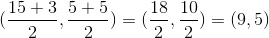This gives a final answer of### Example Question #651 : Equations Of Lines

Find the midpoint of the line segment with the following endpoints: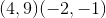Possible Answers: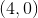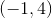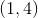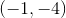Correct answer:Explanation:

Finding the midpoint of a line segment only requires that we find the midpoint, or average of both the x and y components. In order to do that, we use the following formula:For the points (4,9) and (-2,-1) plug in the numbers and solve: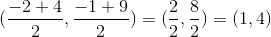This gives a final answer of### Example Question #61 : How To Find The Midpoint Of A Line Segment

Find the midpoint of the line segment with the following endpoints: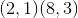Possible Answers:Correct answer:Explanation:

Finding the midpoint of a line segment only requires that we find the midpoint, or average of both the x and y components. In order to do that, we use the following formula:For the points (2,1) and (8,3) plug in the numbers and solve: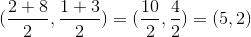This gives a final answer of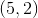### Example Question #61 : How To Find The Midpoint Of A Line Segment

Find the midpoint of the line segment with the following endpoints: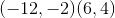Possible Answers: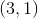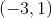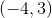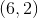Correct answer:Explanation:

Finding the midpoint of a line segment only requires that we find the midpoint, or average of both the x and y components. In order to do that, we use the following formula:For the points (-12,-2) and (6,4) plug in the numbers and solve: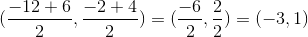This gives a final answer of### Example Question #4181 : Algebra 1

Find the midpoint of the line segment with the following endpoints: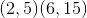Possible Answers: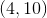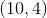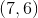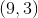Correct answer:Explanation:

Finding the midpoint of a line segment only requires that we find the midpoint, or average of both the x and y components. In order to do that, we use the following formula:For the points (2,5) and (6,15) plug in the numbers and solve: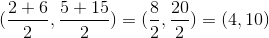This gives a final answer of### Example Question #4182 : Algebra 1

Find the equation of the line segment with the following endpoints: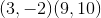Possible Answers: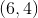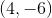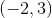Correct answer:Explanation:

Finding the midpoint of a line segment only requires that we find the midpoint, or average of both the x and y components. In order to do that, we use the following formula:For the points (3,-2) and (9,10) plug in the numbers and solve: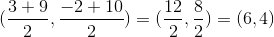This gives a final answer of### All Algebra 1 Resources